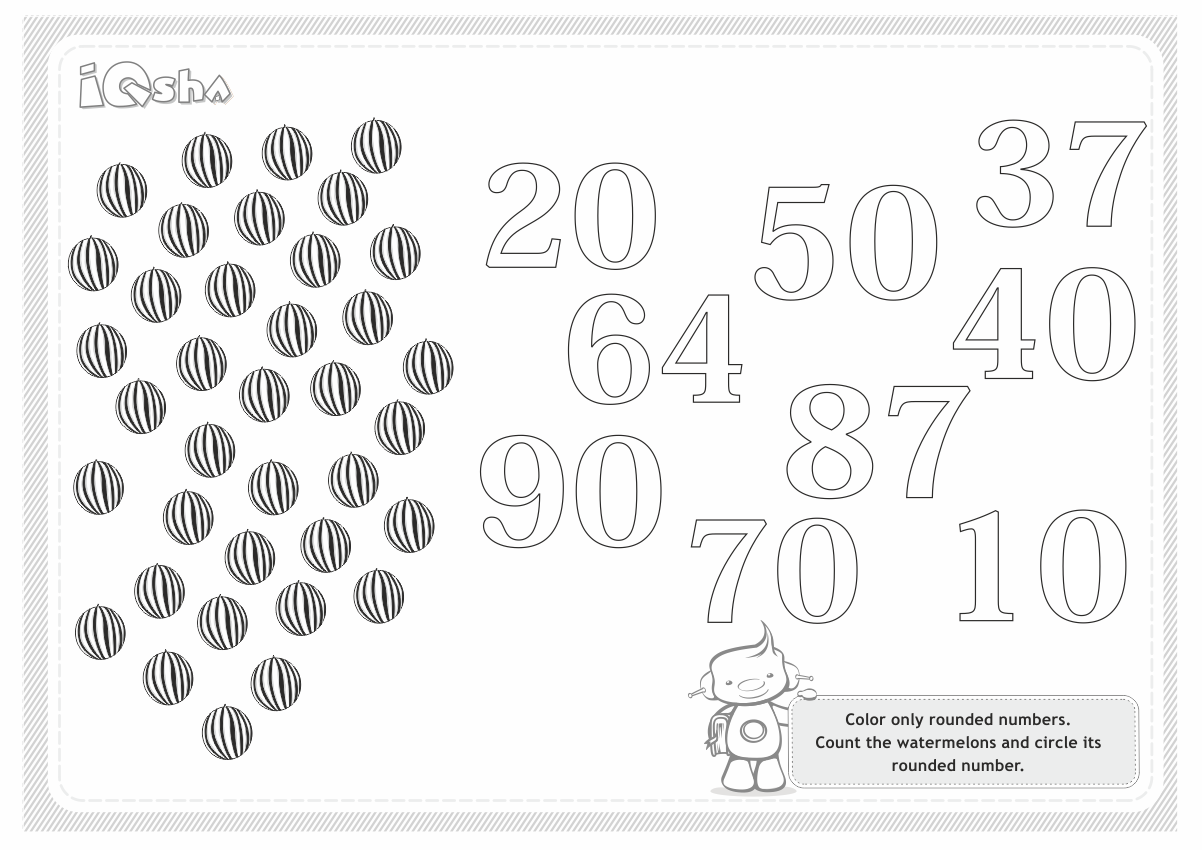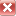# Counting by Tens

24/02/2014, 15:24

How can you count a lot of subjects? Counting one after another, adding one unit at a time, will take a lot of time. If your child has already learned to count up to 10 and higher, it is possible to teach him how to count in tens up to 100 (one hundred).

• Study the names of tens. Scatter a hundred calculating sticks or a hundred office paper clips, a hundred of buttons, or a hundred pieces of twisted macaroni on the table. How do you know if there is exactly one hundred? You can divide all the subjects into many small groups of 10 pieces. Ask the child how many tens are in the first small group? There are 10 subjects, but if you’re talking about tens, there is only one. And how many in two groups? There are two tens, but TWENTY subjects. Enumerate the names of all the tens and check your child’s comprehension by asking the following questions:

- What do we call 7 tens?

- How many tens are there in 50?

- And what about 13? How many tens does it include?

-Do you remember how many subjects there were? How many tens do we have? How many tens are in 100?For the second lesson, diregard the difference between the names of the numbers of the second tens (thirteen, fifteen) and names of the tens (thirty, fifty)– it is difficult to remember the similar words.

• Round and "not round" tens.  Ask the child how to write down the number ‘two’. What about how to write down the number ‘three’, if the ‘tens’ is 20? It is necessary to encourage your child to do this task properly until he can write down or show the number 23. ‘Twenty-three’ is not two plus three, and the number ‘eleven’ is not one plus one! Tell the child or remind him again about two-digit numbers – numbers you write down with the help of 2 numbers. Also, explain that tens can be round (written with zero on the right) and not round numbers.

If you Like it, Share it :Sums with Clothespins

It is difficult for some children to do exercises involving addition and subtraction using a sheet of paper, especially if its not something they study often. It helps to train their skills of mental arithmetic using hand made items.